Miscellaneous

Chapter 10 Class 12 Vector Algebra
Serial order wise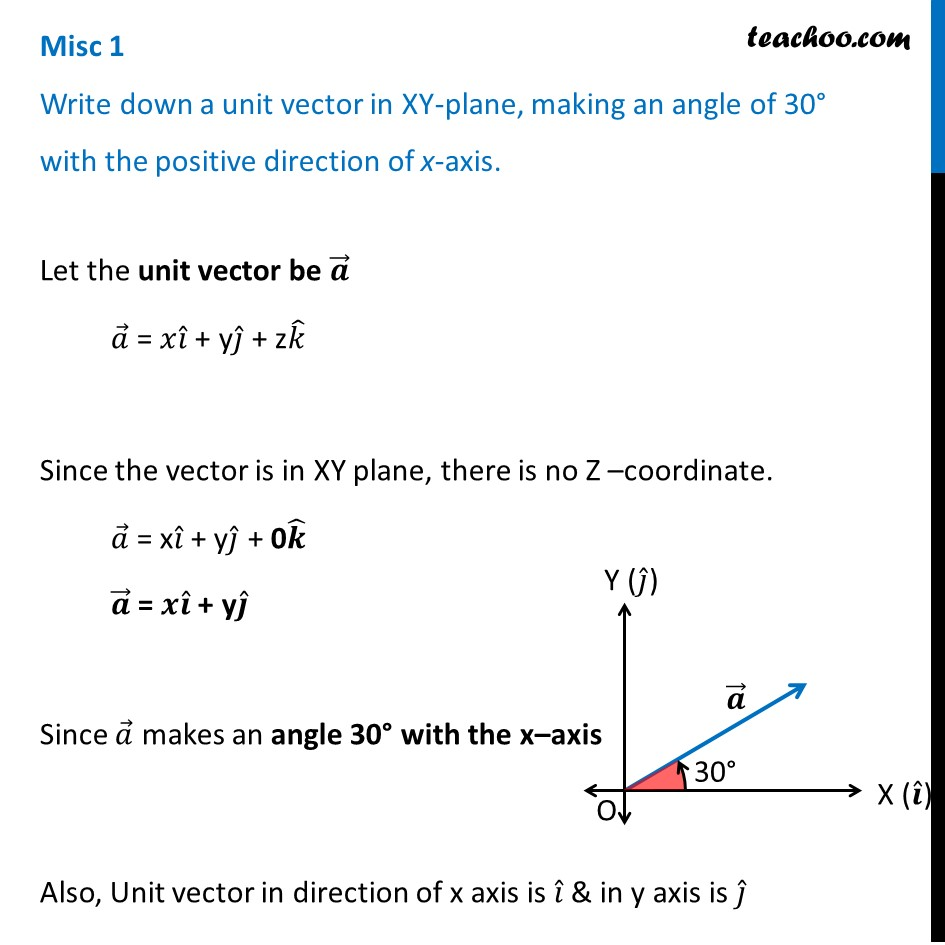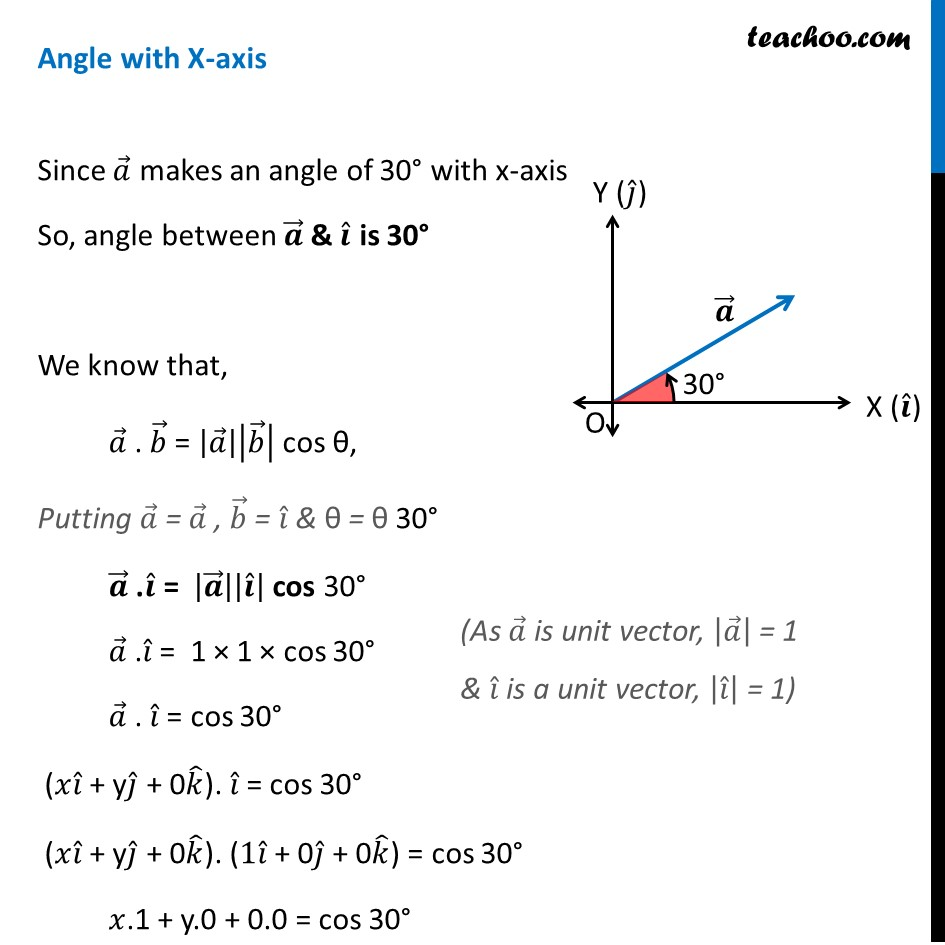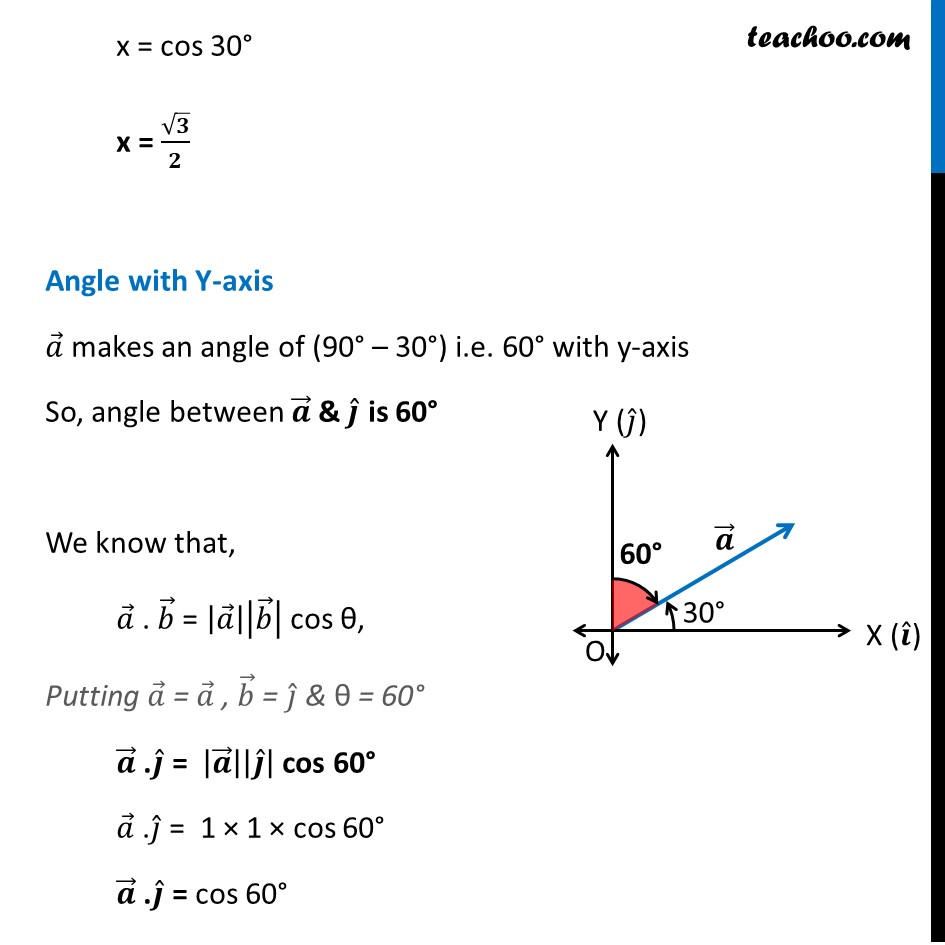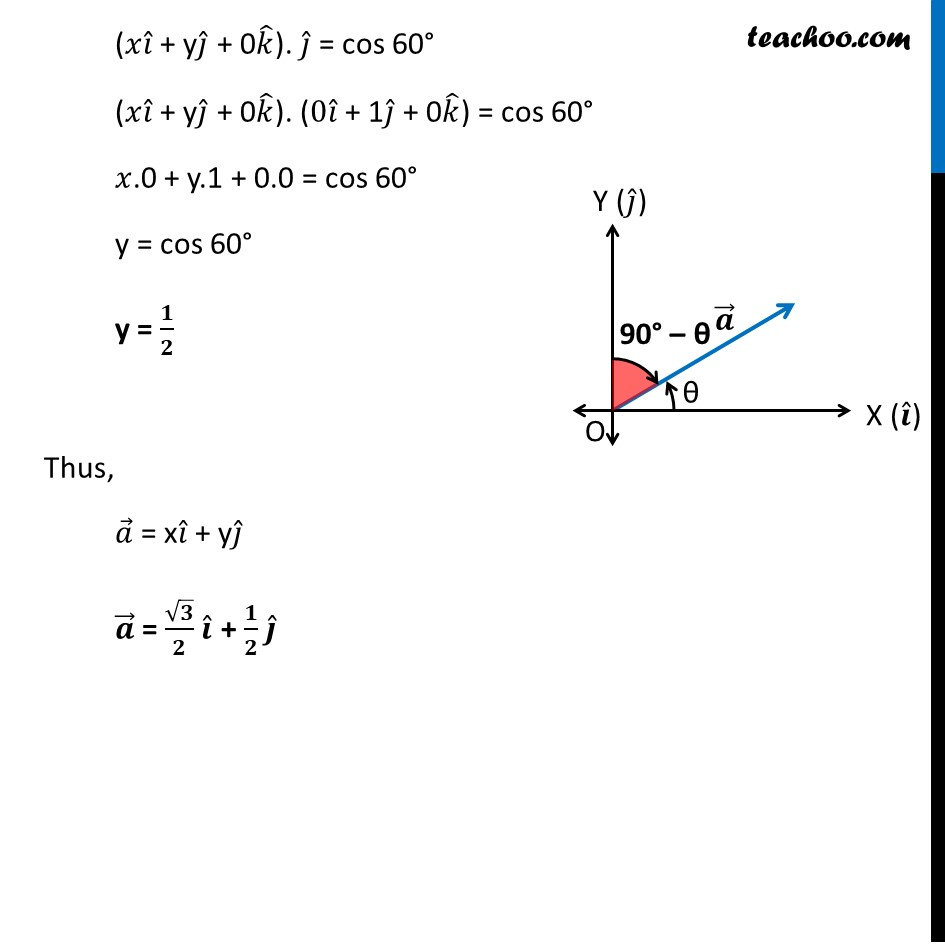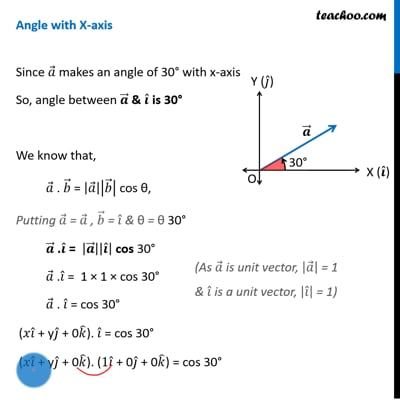This video is only available for Teachoo black users

Learn in your speed, with individual attention - Teachoo Maths 1-on-1 Class

### Transcript

Misc 1 Write down a unit vector in XY-plane, making an angle of 30° with the positive direction of x-axis.Let the unit vector be 𝒂 ⃗ 𝑎 ⃗ = 𝑥𝑖 ̂ + y𝑗 ̂ + z𝑘 ̂ Since the vector is in XY plane, there is no Z –coordinate. 𝑎 ⃗ = x𝑖 ̂ + y𝑗 ̂ + 0𝒌 ̂ 𝒂 ⃗ = 𝒙𝒊 ̂ + y𝒋 ̂ Since 𝑎 ⃗ makes an angle 30° with the x–axis Also, Unit vector in direction of x axis is 𝑖 ̂ & in y axis is 𝑗 ̂ Angle with X-axis Since 𝑎 ⃗ makes an angle of 30° with x-axis So, angle between 𝒂 ⃗ & 𝒊 ̂ is 30° We know that, 𝑎 ⃗ . 𝑏 ⃗ = |𝑎 ⃗ ||𝑏 ⃗ | cos θ, Putting 𝑎 ⃗ = 𝑎 ⃗ , 𝑏 ⃗ = 𝑖 ̂ & θ = θ 30° 𝒂 ⃗ .𝒊 ̂ = |𝒂 ⃗ ||𝒊 ̂ | cos 30° 𝑎 ⃗ .𝑖 ̂ = 1 × 1 × cos 30° 𝑎 ⃗ . 𝑖 ̂ = cos 30° (𝑥𝑖 ̂ + y𝑗 ̂ + 0𝑘 ̂). 𝑖 ̂ = cos 30° (𝑥𝑖 ̂ + y𝑗 ̂ + 0𝑘 ̂). (1𝑖 ̂ + 0𝑗 ̂ + 0𝑘 ̂) = cos 30° 𝑥.1 + y.0 + 0.0 = cos 30° (As 𝑎 ⃗ is unit vector, |𝑎 ⃗ | = 1 & 𝑖 ̂ is a unit vector, |𝑖 ̂ | = 1) x = cos 30° x = √𝟑/𝟐 Angle with Y-axis 𝑎 ⃗ makes an angle of (90° – 30°) i.e. 60° with y-axis So, angle between 𝒂 ⃗ & 𝒋 ̂ is 60° We know that, 𝑎 ⃗ . 𝑏 ⃗ = |𝑎 ⃗ ||𝑏 ⃗ | cos θ, Putting 𝑎 ⃗ = 𝑎 ⃗ , 𝑏 ⃗ = 𝑗 ̂ & θ = 60° 𝒂 ⃗ .𝒋 ̂ = |𝒂 ⃗ ||𝒋 ̂ | cos 60° 𝑎 ⃗ .𝑗 ̂ = 1 × 1 × cos 60° 𝒂 ⃗ .𝒋 ̂ = cos 60° (𝑥𝑖 ̂ + y𝑗 ̂ + 0𝑘 ̂). 𝑗 ̂ = cos 60° (𝑥𝑖 ̂ + y𝑗 ̂ + 0𝑘 ̂). (0𝑖 ̂ + 1𝑗 ̂ + 0𝑘 ̂) = cos 60° 𝑥.0 + y.1 + 0.0 = cos 60° y = cos 60° y = 𝟏/𝟐 Thus, 𝑎 ⃗ = x𝑖 ̂ + y𝑗 ̂ 𝒂 ⃗ = √𝟑/𝟐 𝒊 ̂ + 𝟏/𝟐 𝒋 ̂